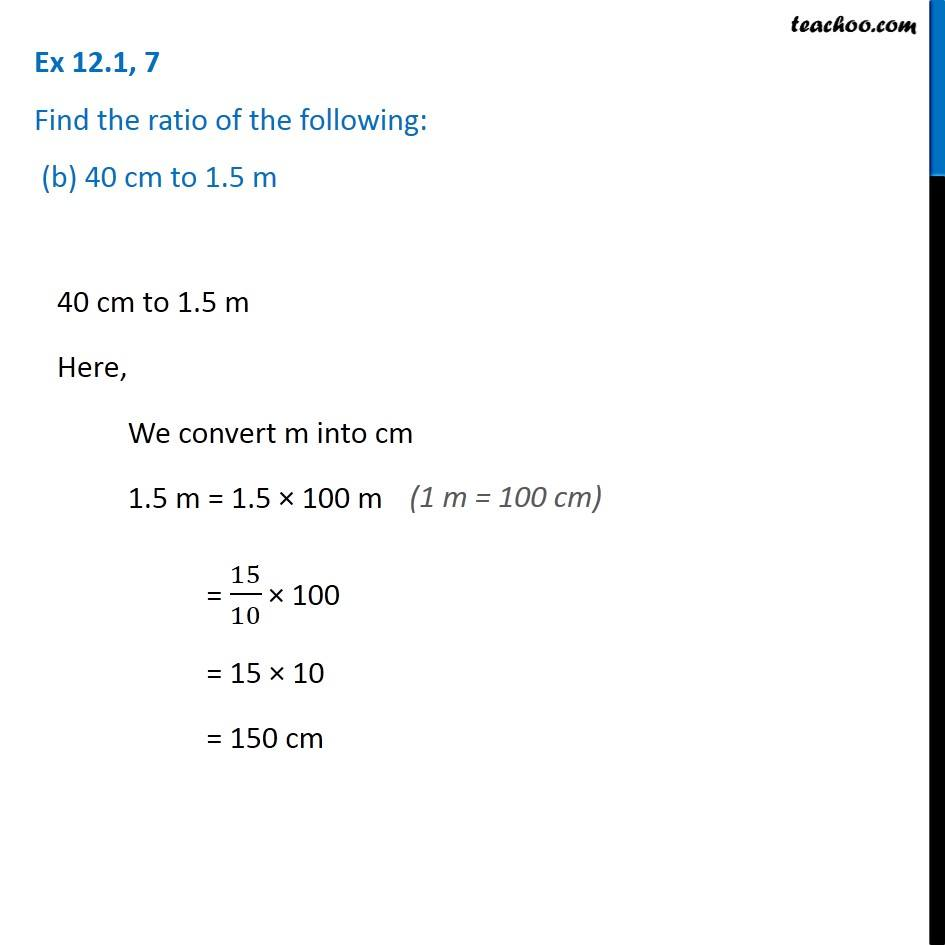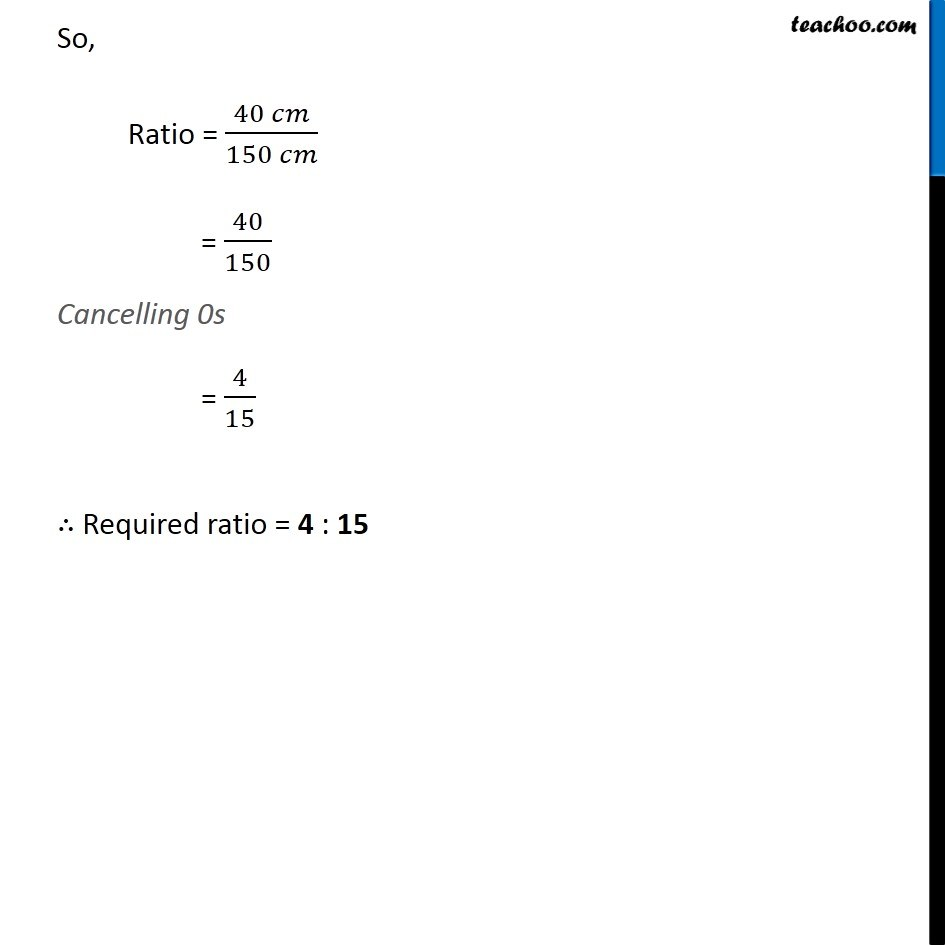Ex 12.1

Chapter 12 Class 6 Ratio and Proportion
Serial order wiseLearn in your speed, with individual attention - Teachoo Maths 1-on-1 Class

### Transcript

Ex 12.1, 7 Find the ratio of the following: (b) 40 cm to 1.5 m40 cm to 1.5 m Here, We convert m into cm 1.5 m = 1.5 × 100 m = 15/10 × 100 = 15 × 10 = 150 cm So, Ratio = (40 𝑐𝑚)/(150 𝑐𝑚) = 40/150 Cancelling 0s = 4/15 ∴ Required ratio = 4 : 15